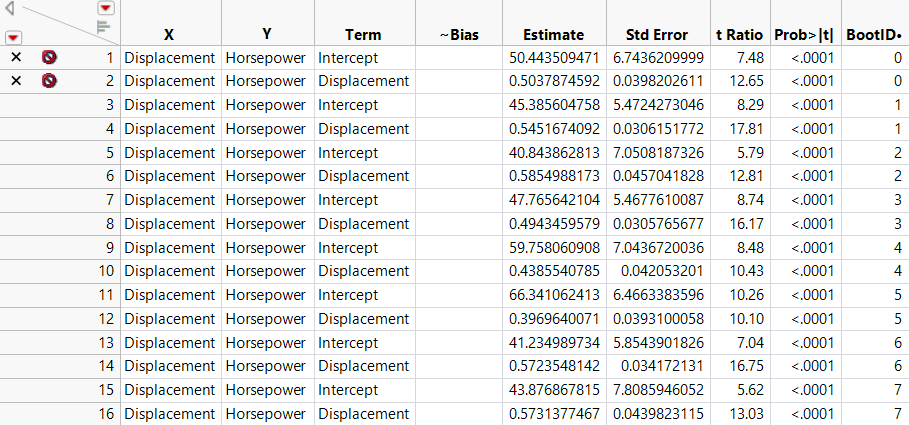Basic Analysis > Bootstrapping > Stacked Results Table
Publication date: 08/13/2020

#Stacked Results Table

The initial results of a bootstrap analysis appear in a stacked results table (Figure 11.4). This table might not appear if you have selected the Discard Stacked Table if Split Works option. Figure 11.4 shows a bootstrap table that is based on the Parameter Estimates report obtained by fitting a Bivariate model in Fit Y by X to Car Physical Data.jmp. See Overview of Bootstrapping.

Figure 11.4 Stacked Bootstrap Results TableNote the following about the stacked results table:

For each bootstrap sample, there is a row for each value given in the first column of the report table. These values are shown in a column whose name is the name of the first column in the report table. In this example, for each bootstrap sample there is a row containing results for each Term: Intercept and Displacement, which appear in the Term column.

The data table columns that are used in the analysis appear in the table. In this example, X is Displacement, and Y is Horsepower.

There is a column for every column in the report table that you are bootstrapping. In this example, the columns are ~Bias, Estimate, Std Error, t Ratio, and Prob>|t|. Note that ~Bias is a column in the Fit Y by X report that is hidden unless one of the parameter estimates is biased.

The BootID• column identifies the bootstrap sample. The rows where BootID• = 0 correspond to the original estimates. Those rows are marked with an X and have the excluded row state. In this example, each bootstrap sample is used to calculate results for two rows: the results for Intercept and the results for Displacement.

The data table name begins with “Stacked Bootstrap Results”.

If you selected the Split Selected Column option, an unstacked results table might also appear. See Unstacked Bootstrap Results Table.